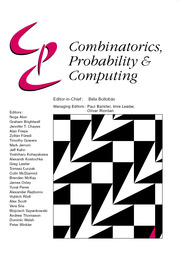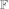Skip to main content Accessibility help
Home
Hostname: page-component-5cfd469876-ws85r Total loading time: 0.307 Render date: 2021-06-24T12:54:12.660Z Has data issue: true Feature Flags: { "shouldUseShareProductTool": true, "shouldUseHypothesis": true, "isUnsiloEnabled": true, "metricsAbstractViews": false, "figures": true, "newCiteModal": false, "newCitedByModal": true, "newEcommerce": true }Combinatorics, Probability and Computing

# Testing Odd-Cycle-Freeness in Boolean Functions

Published online by Cambridge University Press:  10 August 2012

Corresponding
E-mail address:

## Abstract

A function f:2n → {0,1} is odd-cycle-free if there are no x1,. . .,xk2n with k an odd integer such that f(x1) = ··· = f(xk) = 1 and x1 + ··· + xk = 0. We show that one can distinguish odd-cycle-free functions from those ε-far from being odd-cycle-free by making poly(1/ε) queries to an evaluation oracle. We give two proofs of this result, each shedding light on a different connection between testability of properties of Boolean functions and of dense graphs.

The first issue we study is directly reducing testing of linear-invariant properties of Boolean functions to testing associated graph properties. We show a black-box reduction from testing odd-cycle-freeness to testing bipartiteness of graphs. Such reductions have already been shown (Král’, Serra and Vena, and Shapira) for monotone linear-invariant properties defined by forbidding solutions to a finite number of equations. But for odd-cycle-freeness whose description involves an infinite number of forbidden equations, a reduction to graph property testing was not previously known. If one could show such a reduction more generally for any linear-invariant property closed under restrictions to subspaces, then it would likely lead to a characterization of the one-sided testable linear-invariant properties, an open problem raised by Sudan.

The second issue we study is whether there is an efficient canonical tester for linear-invariant properties of Boolean functions. A canonical tester for linear-invariant properties operates by picking a random linear subspace and then checking whether the restriction of the input function to the subspace satisfies a fixed property. The question is if, for every linear-invariant property, there is a canonical tester for which there is only a polynomial blow-up from the optimal query complexity. We answer the question affirmatively for odd-cycle-freeness. The general question remains open.

## Keywords

Type
Paper
Information
Combinatorics, Probability and Computing , November 2012 , pp. 835 - 855
Copyright
Copyright © Cambridge University Press 2012

## Access options

Get access to the full version of this content by using one of the access options below.

## References

Alon, N. (2002) Testing subgraphs in large graphs. Random Struct. Alg. 21 359370.CrossRefGoogle Scholar
Alon, N., Duke, R. A., Lefmann, H., Rödl, V. and Yuster, R. (1994) The algorithmic aspects of the regularity lemma. J. Algorithms 16 80109.CrossRefGoogle Scholar
Alon, N., Fernandez de la Vega, W., Kannan, R. and Karpinski, M. (2003) Random sampling and approximation of MAX-CSPs. J. Comput. Syst. Sci. 67 212243.CrossRefGoogle Scholar
Alon, N., Fischer, E., Krivelevich, M. and Szegedy, M. (2000) Efficient testing of large graphs. Combinatorica 20 451476.CrossRefGoogle Scholar
Alon, N., Kaufman, T., Krivelevich, M., Litsyn, S. and Ron, D. (2005) Testing Reed–Muller codes. IEEE Trans. Inform. Theory 51 40324039.CrossRefGoogle Scholar
Alon, N. and Krivelevich, M. (2002) Testing k-colorability. SIAM J. Discrete Math. 15 211227.CrossRefGoogle Scholar
Alon, N. and Shapira, A. (2008) A characterization of the (natural) graph properties testable with one-sided error. SIAM J. Comput. 37 17031727.CrossRefGoogle Scholar
Austin, T. and Tao, T. (2010) Testability and repair of hereditary hypergraph properties. Random Struct. Alg. 36 373463.Google Scholar
Bhattacharyya, A., Chen, V., Sudan, M. and Xie, N. (2011) Testing linear-invariant non-linear properties. Theory of Computing 7 75–99. Earlier version in STACS ‘09.CrossRefGoogle Scholar
Bhattacharyya, A., Grigorescu, E., Raghavendra, P. and Shapira, A. (2012) Testing odd-cycle-freeness in Boolean functions. In Proc. ACM–SIAM Symposium on Discrete Algorithms, pp. 1140–1149.CrossRefGoogle Scholar
Bhattacharyya, A., Grigorescu, E. and Shapira, A. (2010) A unified framework for testing linear-invariant properties. In Proc. 51st Annual IEEE Symposium on Foundations of Computer Science, IEEE Computer Society, pp. 478487.Google Scholar
Bhattacharyya, A. and Xie, N. (2010) Lower bounds for testing triangle-freeness in Boolean functions. In Proc. 21st ACM–SIAM Symposium on Discrete Algorithms, pp. 87–98.CrossRefGoogle Scholar
Blum, M., Luby, M. and Rubinfeld, R. (1993) Self-testing/correcting with applications to numerical problems. J. Comput. Syst. Sci. 47 549595. Earlier version in STOC'90.CrossRefGoogle Scholar
Bogdanov, A. and Trevisan, L. (2004) Lower bounds for testing bipartiteness in dense graphs. In Proc. 19th Annual IEEE Conference on Computational Complexity, IEEE Computer Society, pp. 7581.Google Scholar
Chen, V., Sudan, M. and Xie, N. (2011) Property testing via set-theoretic operations. In Proc. 2nd Innovations in Computer Science, pp. 211–222.Google Scholar
Goldreich, O., Goldwasser, S. and Ron, D. (1998) Property testing and its connection to learning and approximation. J. Assoc. Comput. Mach. 45 653750.CrossRefGoogle Scholar
Goldreich, O. and Ron, D. (2011) Algorithmic aspects of property testing in the dense graphs model. SIAM J. Comput. 40 376445.CrossRefGoogle Scholar
Goldreich, O. and Trevisan, L. (2003) Three theorems regarding testing graph properties. Random Struct. Alg. 23 2357.CrossRefGoogle Scholar
Gopalan, P., O'Donnell, R., Servedio, R. A., Shpilka, A. and Wimmer, K. (2009) Testing Fourier dimensionality and sparsity. In Proc. 36th Annual International Conference on Automata, Languages, and Programming, Springer, pp. 500512.CrossRefGoogle Scholar
Green, B. (2005) A Szemerédi-type regularity lemma in abelian groups. Geometric Funct. Anal. 15 340376.CrossRefGoogle Scholar
Kaufman, T., Krivelevich, M. and Ron, D. (2004) Tight bounds for testing bipartiteness in general graphs. SIAM J. Comput. 33 14411483.CrossRefGoogle Scholar
Kaufman, T. and Sudan, M. (2008) Algebraic property testing: The role of invariance. In Proc. 40th Annual ACM Symposium on the Theory of Computing, pp. 403–412.CrossRefGoogle Scholar
Král’, D., Serra, O. and Vena, L. (2012) A removal lemma for systems of linear equations over finite fields. Israel J. Math. 187 193207.CrossRefGoogle Scholar
Nagle, B., Rödl, V. and Schacht, M. (2006) The counting lemma for regular k-uniform hypergraphs. Random Struct. Alg. 28 113179.CrossRefGoogle Scholar
Parnas, M., Ron, D. and Rubinfeld, R. (2006) Tolerant property testing and distance approximation. J. Comput. Syst. Sci. 72 10121042.CrossRefGoogle Scholar
Rödl, V. and Schacht, M. (2009) Generalizations of the removal lemma. Combinatorica 29 467502.CrossRefGoogle Scholar
Rödl, V. and Skokan, J. (2004) Regularity lemma for k-uniform hypergraphs. Random Struct. Alg. 25 142.CrossRefGoogle Scholar
Rubinfeld, R. and Sudan, M. (1996) Robust characterizations of polynomials with applications to program testing. SIAM J. Comput. 25 252271.CrossRefGoogle Scholar
Ruzsa, I. and Szemerédi, E. (1978) Triple systems with no six points carrying three triangles. In Combinatorics: Keszthely 1976, Vol. 18 of Colloq. Math. Soc. János Bolyai, North-Holland, pp. 939945.Google Scholar
Shapira, A. (2009) Green's conjecture and testing linear-invariant properties. In Proc. 41st Annual ACM Symposium on the Theory of Computing, pp. 159–166.CrossRefGoogle Scholar
Sudan, M. (2010) Invariance in property testing. In Property Testing: Current Research and Surveys (Goldreich, O., ed.), Vol. 6390 of Lecture Notes in Computer Science, Springer, pp. 211227.CrossRefGoogle Scholar
4
Cited by

# Send article to Kindle

To send this article to your Kindle, first ensure no-reply@cambridge.org is added to your Approved Personal Document E-mail List under your Personal Document Settings on the Manage Your Content and Devices page of your Amazon account. Then enter the ‘name’ part of your Kindle email address below. Find out more about sending to your Kindle. Find out more about sending to your Kindle.

Note you can select to send to either the @free.kindle.com or @kindle.com variations. ‘@free.kindle.com’ emails are free but can only be sent to your device when it is connected to wi-fi. ‘@kindle.com’ emails can be delivered even when you are not connected to wi-fi, but note that service fees apply.

Find out more about the Kindle Personal Document Service.

Testing Odd-Cycle-Freeness in Boolean Functions
Available formats
×

# Send article to Dropbox

To send this article to your Dropbox account, please select one or more formats and confirm that you agree to abide by our usage policies. If this is the first time you use this feature, you will be asked to authorise Cambridge Core to connect with your <service> account. Find out more about sending content to Dropbox.

Testing Odd-Cycle-Freeness in Boolean Functions
Available formats
×

# Send article to Google Drive

To send this article to your Google Drive account, please select one or more formats and confirm that you agree to abide by our usage policies. If this is the first time you use this feature, you will be asked to authorise Cambridge Core to connect with your <service> account. Find out more about sending content to Google Drive.

Testing Odd-Cycle-Freeness in Boolean Functions
Available formats
×
×

#### Reply to:Submit a response

Please enter your response.

#### Your details

Please enter a valid email address.

#### Conflicting interests

Do you have any conflicting interests? *